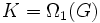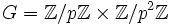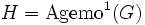# Characteristicity does not satisfy intermediate subgroup condition

This article gives the statement, and possibly proof, of a subgroup property (i.e., characteristic subgroup) not satisfying a subgroup metaproperty (i.e., intermediate subgroup condition).
View all subgroup metaproperty dissatisfactions | View all subgroup metaproperty satisfactions|Get help on looking up metaproperty (dis)satisfactions for subgroup properties
Get more facts about characteristic subgroup|Get more facts about intermediate subgroup condition|

## Statement

It is possible to have a group$G$ with a characteristic subgroup$H$, and a subgroup$K \le G$ containing$H$, such that$H$ is not characteristic as a subgroup of$K$.

## Partial truth

### Corresponding statement for normality

Further information: Normality satisfies intermediate subgroup condition

If$H \le K \le G$ are such that$H$ is normal in$G$, then$H$ is normal in$K$. In particular, since every characteristic subgroup is normal, it follows that if$H$ is characteristic in$G$,$H$ is normal in$K$.

### Intermediately characteristic subgroup

Further information: Intermediately characteristic subgroup

A subgroup of a group that is characteristic in every intermediate subgroup is termed an intermediately characteristic subgroup. Under certain conditions, we can guarantee a subgroup to be intermediately characteristic. For instance, any normal Sylow subgroup, any more generally, any normal Hall subgroup, is intermediately characteristic. There are certain subgroup-defining functions that yield intermediately characteristic subgroups, for instance, the perfect core of any group is intermediately characteristic. These are, however, extremely rare.

### Potentially characteristic subgroup

Further information: NPC theorem

A subgroup$H$ of a group$K$ is termed potentially characteristic if there exists a group$G$ containing$K$ such that$H$ is characteristic in$G$. Clearly, every potentially characteristic subgroup is normal; on the other hand, since characteristicity does not satisfy the intermediate subgroup condition, not every potentially characteristic subgroup is characteristic.

The NPC theorem states that every normal subgroup is potentially characteristic, and the finite NPC theorem proves this in the context of finite groups.

### Subgroup in which every subgroup characteristic in the whole group is characteristic

Further information: Subgroup in which every subgroup characteristic in the whole group is characteristic

A subgroup$K$ of a group$G$ is termed a subgroup in which every subgroup characteristic in the whole group is characteristic if, whenever$H$ is a subgroup of$K$ such that$H$ is characteristic in$G$, then$H$ is characteristic in$K$.

Any direct factor, and more generally, any AEP-subgroup, satisfies this property. Note that characteristic subgroups themselves need not satisfy this property.

## Related facts

### Dual fact

For more on the background, see subgroup-quotient duality for groups.

The dual to this fact is that characteristicity does not satisfy image condition. The duality works as follows:

Notion Dual
characteristic subgroup quotient group by a characteristic subgroup (reframed in terms of the characteristic subgroup itself)
intermediate subgroup condition image condition

Further, if we restrict attention to examples in finite abelian groups, then an example for either can be dualized to an example for other other using the idea that subgroup lattice and quotient lattice of finite abelian group are isomorphic.

## Proof

Note that for any counterexample,$H$ must be a nontrivial subgroup,$K$ must properly contain$H$, and$G$ must properly contain$K$. Thus, the order of$G$ must be at least eight. We give here two counterexamples of size eight: one Abelian, and one non-Abelian.

### Example of an Abelian group of order eight

In the example we construct here, the subgroup$K$ that we construct is also characteristic in$G$.

Consider:

•$G = \mathbb{Z}/2\mathbb{Z} \times \mathbb{Z}/4\mathbb{Z}$.
•$H$ to be the set of squares in$G$, i.e.,$H = \{ (0,0), (0,2) \}$ --$H = \mho^1(G)$ (the first agemo subgroup) and is thus characteristic.
•$K$ to be the set of elements of order dividing two in$G$, i.e.,$K = \{ (0,0), (1,0), (0,2), (1,2) \}$ --$K = \Omega_1(G)$ (the first omega subgroup) and is thus characteristic.

Now,$K$ is an internal direct product of$H$ and the subgroup$L = \{ (0,0), (1,0) \}$, so it is isomorphic to the Klein four-group, and it admits an isomorphism that exchanges the two direct factors$H$ and$L$. Hence,$H$ is not characteristic in$K$.

The analogous example works for any prime$p$. Specifically:$G = \mathbb{Z}/p\mathbb{Z} \times \mathbb{Z}/p^2\mathbb{Z}$.

•$H$ is the set of all$p^{th}$ powers in$G$, i.e.,$H = \{ (0,kp) \mid k \in \{ 0,1,2,\dots,p-1 \} \}$. Equivalently,$H = \operatorname{Agemo}^1(G)$ and is thus characteristic.
•$K$ is the set of all elements of order dividing$p$ in$G$, i.e.,$K = \{ (a,kp) \mid a \in \mathbb{Z}/p\mathbb{Z}, k \in \{ 0,1,2,\dots,p-1 \} \}$. Equivalently,$K = \Omega_1(G)$ and is thus characteristic.

Now,$K$ is an internal direct product of$H$ and the subgroup$L = \{ (a,0) \mid a \in \mathbb{Z}/p\mathbb{Z} \}$, and there is an exchange automorphism of$K$ interchanging$H$ and$L$. Thus,$H$ is not characteristic in$K$.

### Example of the dihedral group

Further information: dihedral group:D8, center of dihedral group:D8, Klein four-subgroups of dihedral group:D8

Consider the dihedral group of order eight, with an element$a$ of order$4$, that plays the role of the rotation, and$x$, that plays the role of the reflection. Then, consider:

•$G$ is the dihedral group
•$H = \{ e, a^2 \}$ is the center of$G$ -- hence it is characteristic in$G$
•$K = \{ e, a^2, x, a^2x \}$ is a subgroup of$G$ isomorphic to the Klein four-group.

Now,$K$ is the internal direct product of$H$ and the subgroup$L = \{e , x \}$, so it is isomorphic to the Klein four-group, and it admits an automorphism that exchanges the two direct factors$H$ and$L$. Hence,$H$ is not characteristic in$K$.

## GAP implementation

### For the Abelian group example

Here is an implementation of the Abelian group case for the group of order eight:

gap> G := DirectProduct(CyclicGroup(2),CyclicGroup(4));
<pc group of size 8 with 3 generators>
gap> H := Agemo(G,2,1);
Group([ f3 ])
gap> K := Omega(G,2,1);
Group([ f1, f3 ])
gap> IsCharacteristicSubgroup(G,H);
true
gap> IsCharacteristicSubgroup(G,K);
true
gap> IsCharacteristicSubgroup(K,H);
false

For the more general version, replace$2$ by a prime$p$ and$4$ by$p^2$ in the code above.

The direct product$\mathbb{Z}/p\mathbb{Z} \times \mathbb{Z}/p^2\mathbb{Z}$ can also be obtained as the second group of order$p^3$; in other words, it can also be defined as:

G := SmallGroup(p^3,2);